# Set Up Automatic Currency Conversion in Excel & Google Sheets

This tutorial demonstrates how to create and automatic currency converter in Excel and Google Sheets.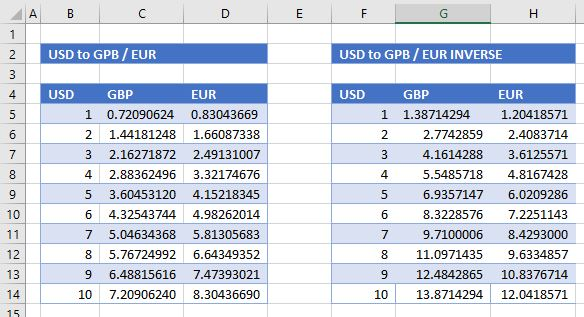Excel is a powerful tool for calculations, often involving international currencies. Excel does not have a built-in currency conversion tool, but with a link to an external XML database, you can build your own conversion tool into Excel using Power Query.

## Link to External XML Source File

The most user-friendly source file is one called Floatrates, a free XML feed that is updated daily.

1. In a blank Excel worksheet, in the Ribbon, go to Data > Get Data > From File > From XML.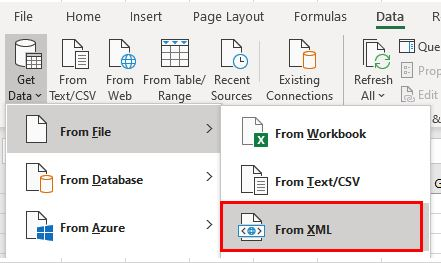1. In the File name field, type in the URL for the Floatrates file – www.floatrates.com/daily.usd.xml – and then click Open.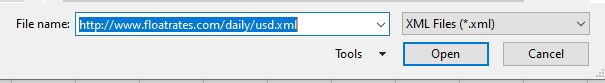1. Click Transform Data.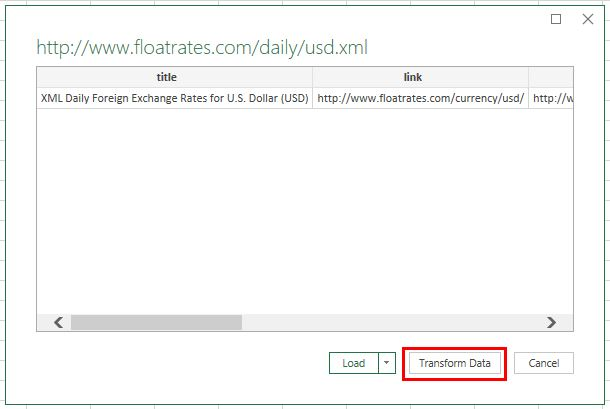1. This opens the Power Query Editor. Scroll across to the last item on the list, and then click the word Table. A table below the initial line of data is populated with the exchange rates.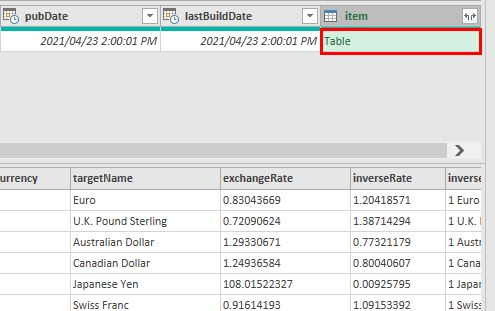1. Click on the double-headed arrow to show the fields of this table, and then click OK.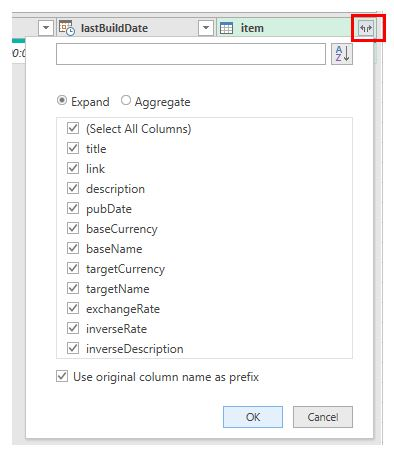1. The Power Query changes to show the exchange rate columns as well as the initial columns.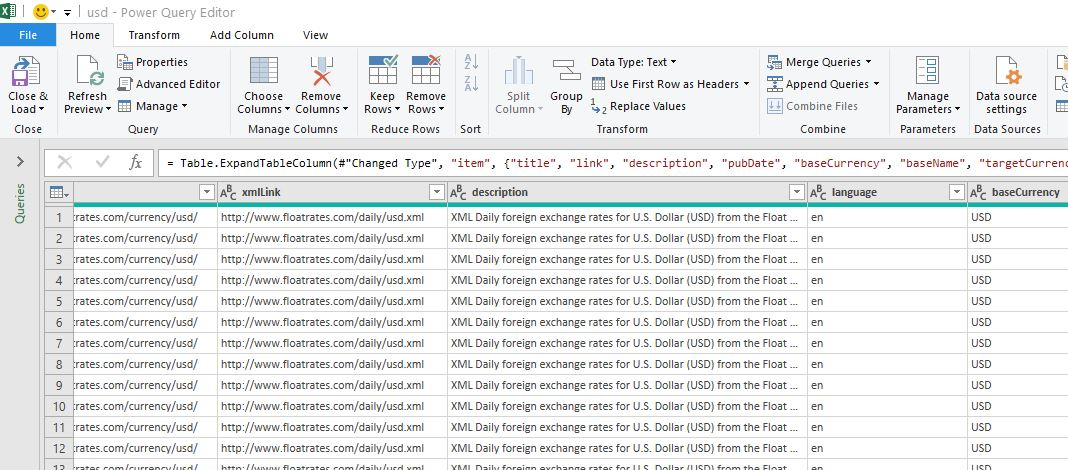1. In the Ribbon, go to Home > Manage Columns > Choose Columns.1. Uncheck the columns you don’t need, and then click OK.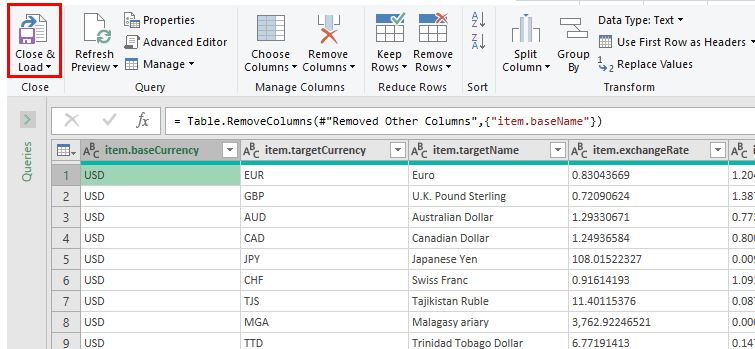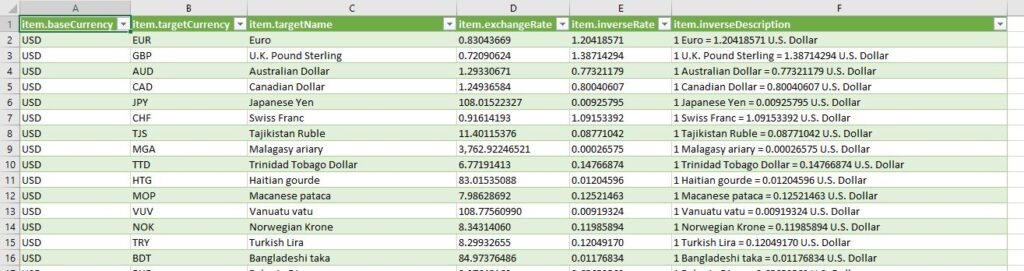## Create Currency Converter

1. Create a new worksheet in the same workbook as the linked XML database and set up a table as shown below.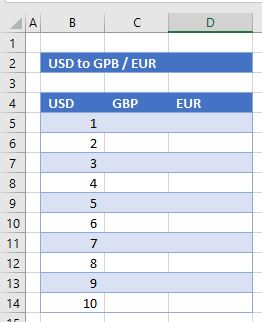1. Use the VLOOKUP Function to look up the currency rate for the British Pound against the US Dollar. In the relevant cell (for example C5), type in:
``=VLOOKUP(\$C\$4,``

Tip: Use an absolute reference (\$) so you can copy the formula down.

1. Then highlight the relevant lookup data by going to the data sheet and selecting the information you need.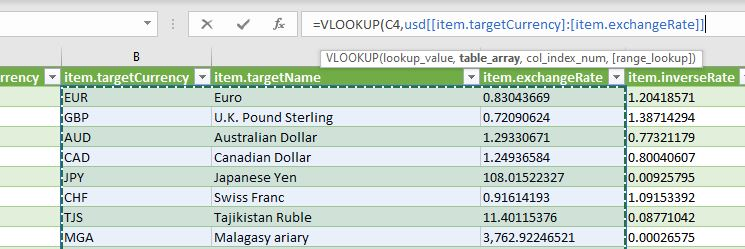1. As soon as you select the entire data list, Excel automatically puts the columns of the database as references in the formula rather than using the cell addresses. This is because the table data in Excel was linked into Excel with a range name of usd.
1. Complete the VLOOKUP formula by typing in the column name and the word FALSE as the final argument for the formula.
``=VLOOKUP(\$C\$4,usd[[item.targetCurrency]:[item.exchangeRate]],3,FALSE)``
1. Press ENTER to enter the formula into Excel.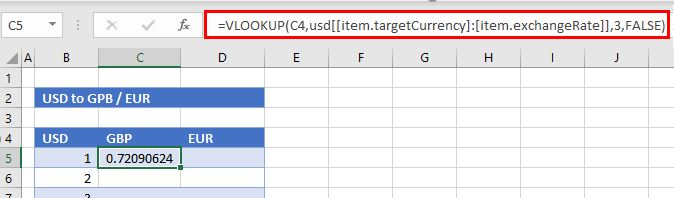1. Repeat the procedure to look up EUR from the data table.
``=VLOOKUP(\$D\$4,usd[[item.targetCurrency]:[item.exchangeRate]],3,FALSE)``
1. To get the value of 2 USD to 1 GBP or 1 EUR, copy the formula to the next row, and then multiply it by the value in Column B.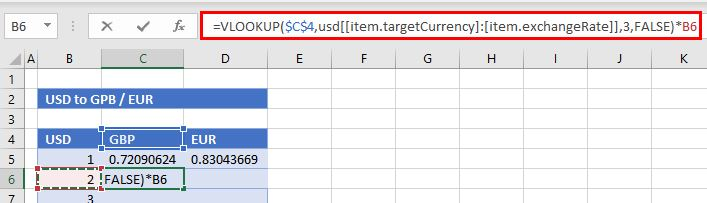1. Copy the formula down to populate the exchange rate converter.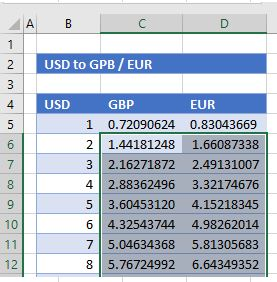1. To create an exchange rate converter for the inverse rates, create another lookup – for the inverse rates in the data.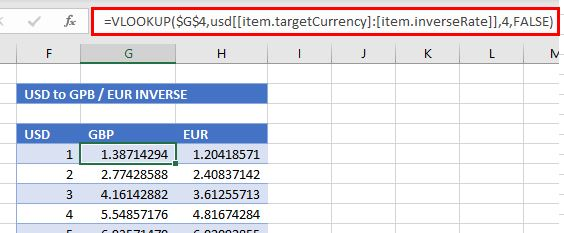## Automatic Currency Conversion in Google Sheets

Google Sheets has a built-in currency converter that makes use of existing up-to-date conversion rates on the internet.

1. Set up a table in Google Sheets similar to the one set up in Excel above.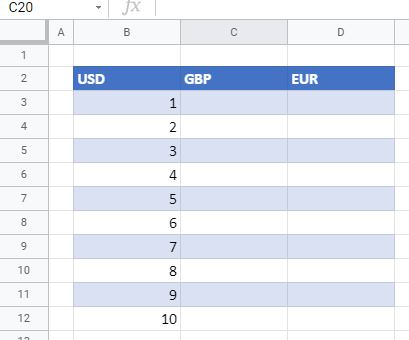1. In the selected cell (for example, C3), type in the formula (with the GOOGLEFINANCE Function):
``=GOOGLEFINANCE("Currency:"&\$B\$2&\$C\$2)``
1. Google automatically looks up the exchange rate from USD (in B2) to GBP (in C2) and fills in the selected cell.1. Since cells B2 and C2 are locked (with \$), you can copy this formula down to the next cell and then multiply the formula by the value in Column B.
``=GOOGLEFINANCE("Currency:"&\$B\$2&\$C\$2)*B4``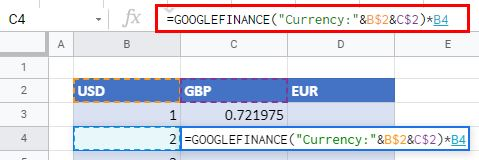1. Copy the formula in this cell down to the remaining rows in the conversion table.
2. Repeat the process to look up the EUR.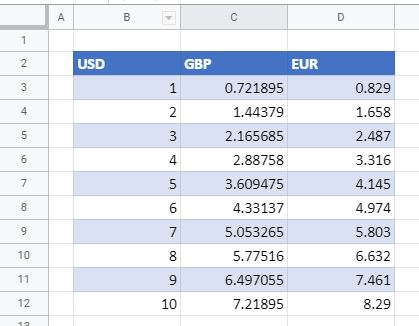Other tutorials that use Power Query